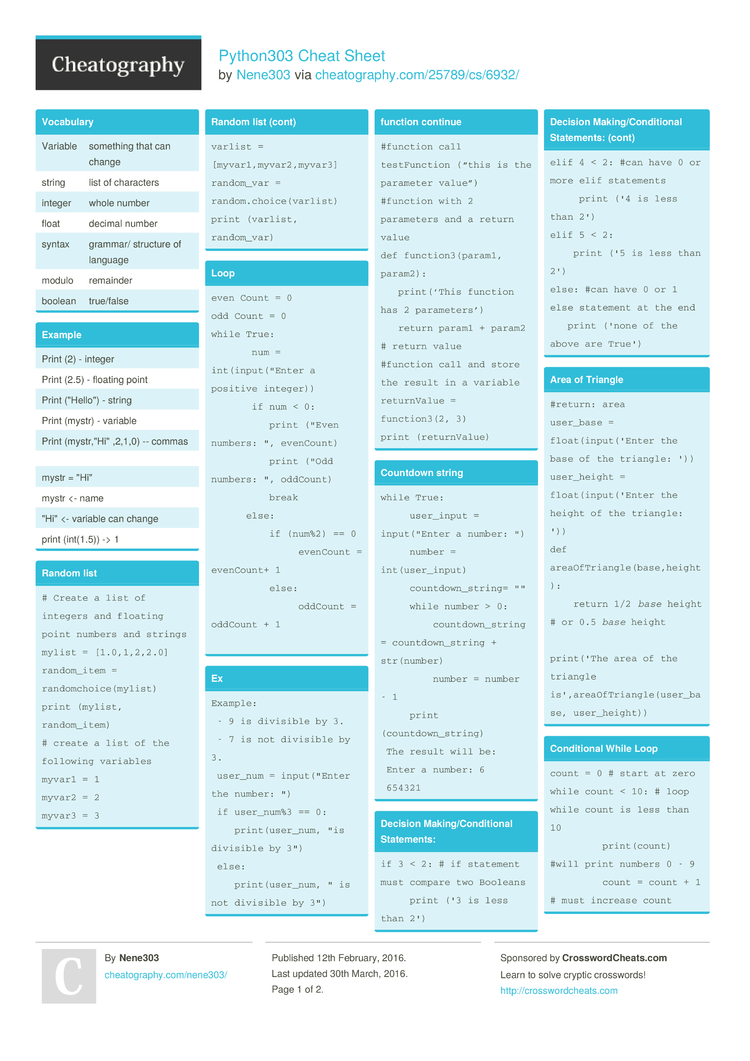Cheatography

# Python303 Cheat Sheet by Nene303

### Vocabulary

 Variable something that can change string list of characters integer whole number float decimal number syntax grammar/ structure of language modulo remainder boolean true/false

### Example

 Print (2) - integer Print (2.5) - floating point Print ("He­llo­") - string Print (mystr) - variable Print (mystr­,"Hi­" ,2,1,0) -- commas mystr = "­Hi" mystr <- name "­Hi" <- variable can change print (int(1.5)) -> 1

### Random list

 ```# Create a list of integers and floating point numbers and strings mylist = [1.0,1,2,2.0] random_item = randomchoice(mylist) print (mylist, random_item) # create a list of the following variables myvar1 = 1 myvar2 = 2 myvar3 = 3 varlist = [myvar1,myvar2,myvar3] random_var = random.choice(varlist) print (varlist, random_var)```

### Loop

 ```even Count = 0 odd Count = 0 while True:        num = int(input("Enter a positive integer))        if num < 0:           print ("Even numbers: ", evenCount)           print ("Odd numbers: ", oddCount)           break       else:           if (num%2) == 0                evenCount = evenCount+ 1           else:                oddCount = oddCount + 1```

### Ex

 ``` Example:  - 9 is divisible by 3.  - 7 is not divisible by 3.  user_num = input("Enter the number: ")  if user_num%3 == 0:     print(user_num, "is divisible by 3")  else:     print(user_num, " is not divisible by 3")```

### function continue

 ```#function call testFunction (“this is the parameter value”) #function with 2 parameters and a return value def function3(param1, param2):    print(‘This function has 2 parameters’)    return param1 + param2 # return value #function call and store the result in a variable returnValue = function3(2, 3) print (returnValue)```

### Countdown string

 ```while True:      user_input = input("Enter a number: ")      number = int(user_input)      countdown_string= ""      while number > 0:          countdown_string = countdown_string + str(number)          number = number - 1      print (countdown_string)  The result will be:  Enter a number: 6  654321```

### Decision Making­/Co­ndi­tional Statem­ents:

 ```if 3 < 2: # if statement must compare two Booleans      print ('3 is less than 2') elif 4 < 2: #can have 0 or more elif statements      print ('4 is less than 2') elif 5 < 2:     print ('5 is less than 2') else: #can have 0 or 1 else statement at the end    print ('none of the above are True')```

### Area of Triangle

 ```#return: area user_base = float(input('Enter the base of the triangle: ')) user_height = float(input('Enter the height of the triangle: ')) def areaOfTriangle(base,height):     return 1/2 base height # or 0.5 base height                      print('The area of the triangle is',areaOfTriangle(user_base, user_height))```

### Condit­ional While Loop

 ```count = 0 # start at zero while count < 10: # loop while count is less than 10          print(count) #will print numbers 0 - 9          count = count + 1 # must increase count```

 ```while True:      user_radius = input("Please enter the radius of the circle: ")      radius = float(user_radius)      pi = 3.1415      area = pi radius * 2      print("The area of the cicle is", area)```2 Pages
//media.cheatography.com/storage/thumb/nene303_python303.750.jpg

PDF (recommended)